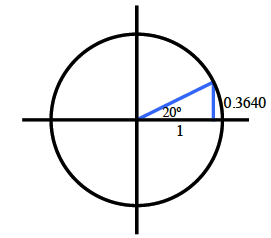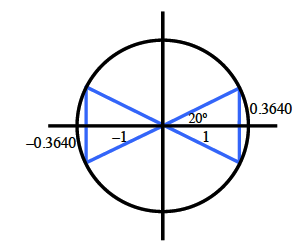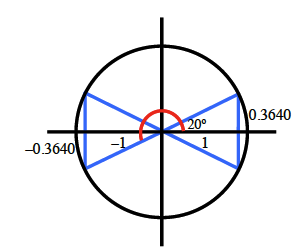### Home > CCA2 > Chapter Ch10 > Lesson 10.2.1 > Problem10-102

10-102.

Use reference angles, the symmetry of a circle, and the knowledge that tan$(20°) ≈ 0.3640$ to write three other true statements using tangent.

Draw the angle and corresponding right triangle in the first quadrant. Recall that tangent is opposite/adjacent, and label the side lengths. Unit circle, right triangle in first quadrant, labeled as follows: vertical leg, 0.3640, angle opposite vertical leg, 20 degrees.

Redraw your triangle in each of the other quadrants. Label the side lengths with the correct $(±)$ sign. Added to unit circle, right triangle in second, third, & fourth quadrants. Triangle in third quadrant, vertical leg labeled, negative 0.3640.

One possible statement: tan$(200°)=\frac{-.3640}{-1}=0.3640$ A small red arc, from the positive, x axis, to the hypotenuse, of the right triangle, in the third quadrant.